# NCERT Solutions For Class 10 Maths Chapter 13 Exercise 13.1

Go back to  'Surface Areas and Volumes'

## Chapter 13 Ex.13.1 Question 1

$$2$$ cubes each of volume $$64 \;\rm{cm}^3$$ are joined end to end. Find the surface area of the resulting cuboid.

### Solution

What is known?

Two cubes each of volume $$64 \;\rm{cm}^3$$  are joined end to end.

What is unknown?

Surface area of the resulting cuboid when two cubes are joined end to end.

Reasoning:

We will find the length of the edge of each cube by using the formula for volume of a cube $$=a^3$$ , where length of the edge is $$a.$$

As the cubes are joined end to end, they will appear as follows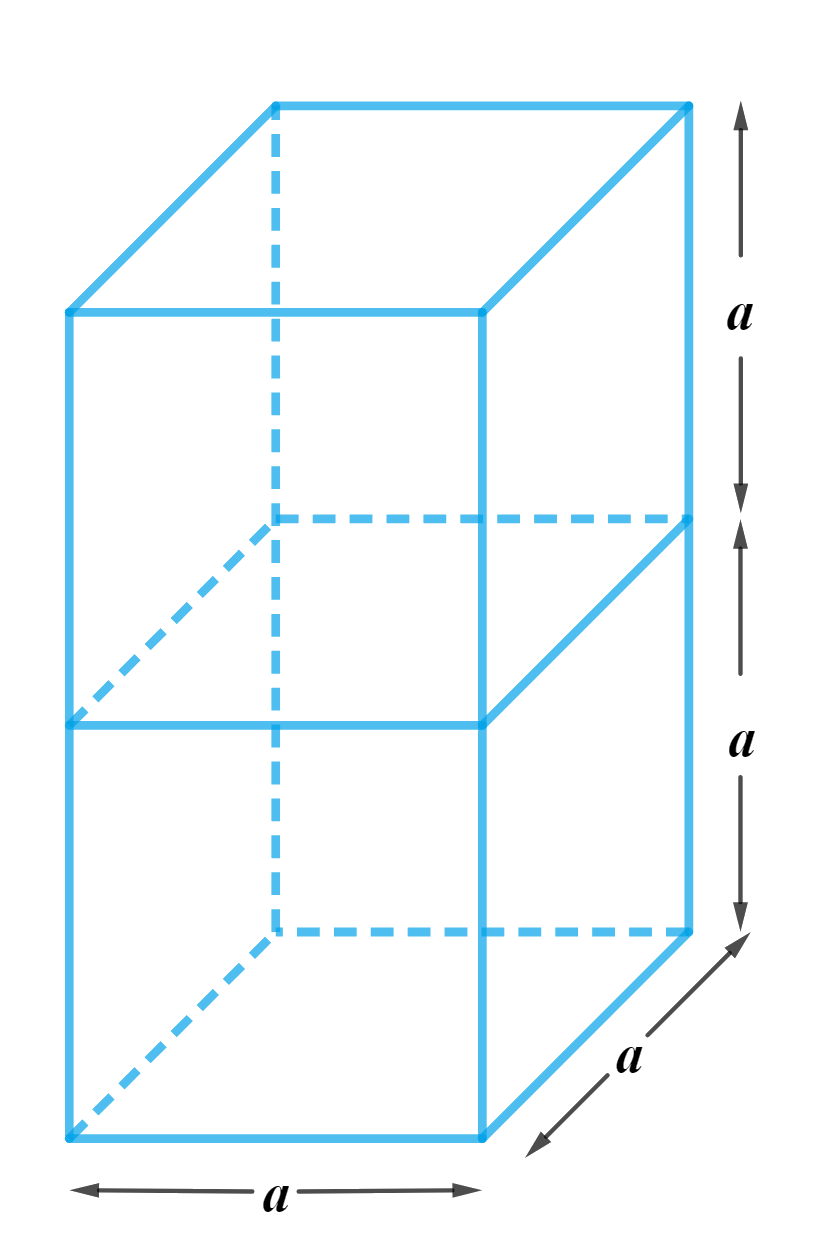Using the formula for Surface area of a cuboid \begin{align} = 2\left( {lb + bh + lh} \right)\end{align} ,

where $$l,b\,\,{\rm{ and }}\,\,h$$  are length, breadth and height respectively. We’ll be able to get the answer.

Steps:

Let the length of the edge of each cube is $$a$$
Therefore, volume of the cube $$=a^3$$

volume of the cube,

\begin{align} {a^3} &= 64 \rm {c{m^3}}\\{a^3} &= 64 \rm {c{m^3}}\\a &= \sqrt{{64 \rm c{m^3}}}\\a &= \sqrt{{{{\left( {4 \rm {cm}} \right)}^3}}}\\a &= 4 \rm {cm}\end{align}

Therefore,

Length of the resulting cuboid,$$l = a = 4\rm{cm}$$
Breadth of the resulting cuboid,$$b = a = 4\rm{cm}$$
Height of the resulting cuboid,$$h = 2a = 2 \times 4\rm{cm} = 8\rm{cm}$$

Surface area of the resulting cuboid

\begin{align} &= 2\left( {lb + bh + lh} \right) \\&= 2 ( \rm 4{cm}\! \times\! 4{cm}\! +\! 4{cm}\! \times\! 8 \rm {cm} \!+ \!4{cm} \!\times\! 8{cm} ) \\&= 2 (16 \rm {c{m^2}} + 32{c{m^2}} + 32 \rm {c{m^2}}) \\\ &= 2 \times 80 \rm {c{m^2}}\\&= 160{\rm c{m^2}}\end{align}

## Chapter 13 Ex.13.1 Question 2

A vessel is in the form of a hollow hemisphere mounted by a hollow cylinder. The diameter of the hemisphere is $$14\;\rm{cm}$$ and the total height of the vessel is $$13 \;\rm{cm}$$. Find the inner surface area of the vessel.

### Solution

What is  known?

The diameter of the hemisphere is $$14\rm{ cm}$$ and total height of the vessel is $$13\rm {cm.}$$

What is unknown?

The inner surface area of the vessel.

Reasoning:

Create a figure of the vessel according to the given description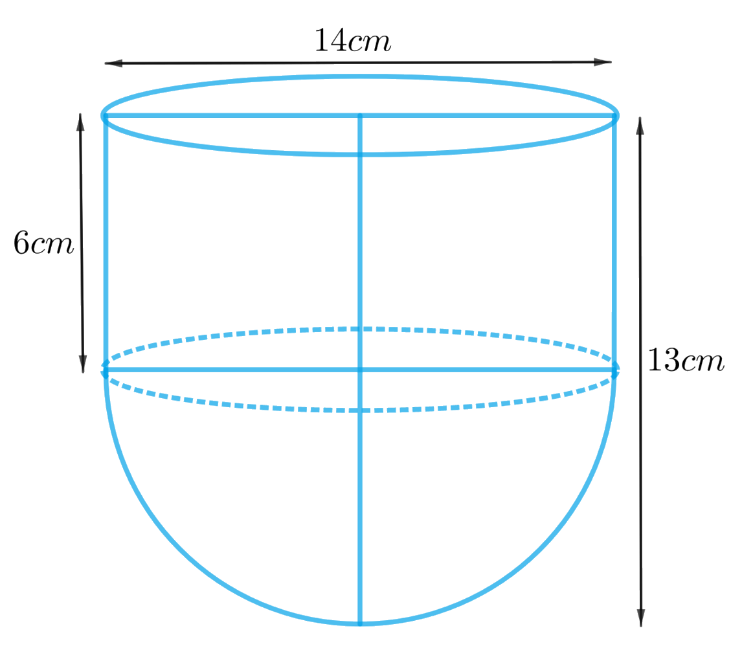From the figure it’s clear that the inner surface area of the vessel includes the CSA of the hemisphere and the cylinder.

Inner surface area of the vessel $$=$$ CSA of the hemisphere $$+$$ CSA of the cylinder

We will find the area of the vessel by using formulae;

CSA of the hemisphere  $$= 2\pi {r^2}$$
where $$r$$ is the radius of the hemisphere

CSA of the cylinder $$= 2\pi rh$$
where $$r$$ and $$h$$ are the radius and height of the cylinder respectively.

Height of the cylinder $$=$$ Total height of the vessel $$–$$ height of the hemisphere

Steps:

Diameter of the hemisphere,$$d = 14 \rm cm$$
Radius of the hemisphere,  \begin{align}r = \frac{{14 \rm cm}}{2} = 7\rm{cm}\end{align}
Height of the hemisphere $$=$$ radius of the hemisphere, $$r = 7 \rm cm$$

Radius of the cylinder,$$r = 7 \rm cm$$

Height of the cylinder $$=$$ Total height of the vessel $$–$$ height of the hemisphere

$h = 13 \rm cm - 7cm = 6cm$

Inner surface area of the vessel $$=$$ CSA of the hemisphere $$+$$ CSA of the cylinder

\begin{align}&= 2\pi {r^2} + 2\pi rh\\&= 2\pi r\left( {r + h} \right)\\&= 2 \times \frac{{22}}{7} \times 7\rm{cm} \left( {7{cm} + 6{cm}} \right)\\&= 2 \times 22 \times 13 \rm {c{m^2}}\\&= 572 \rm {c{m^2}}\end{align}

## Chapter 13 Ex.13.1 Question 3

A toy is in the form of a cone of radius $$3.5\; \rm{cm}$$ mounted on a hemisphere of same radius. The total height of the toy is $$15.5 \;\rm{cm}$$. Find the total surface area of the toy.

### Solution

What is known?

The toy is in the form of a cone of radius $$=3.5\, \rm{cm}$$  mounted on a hemisphere with the same radius. The total height of the toy is $$15.5 \,\rm{cm}$$.

What is unknown?

The total surface area of the toy.

Reasoning:

We can create the figure of the toy as per given information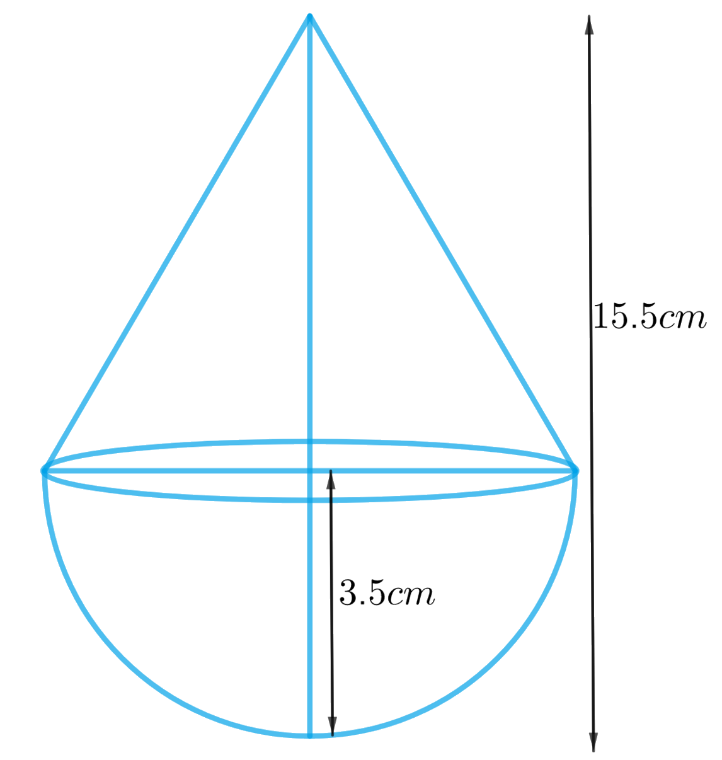From the figure it’s clear that total surface area of the toy includes CSA of the cone and hemisphere.
Total surface area of the toy $$=$$ CSA of the hemisphere $$+$$ CSA of the cone

We will find the total area of the toy by using formulae;
CSA of the hemisphere $$= 2\pi {r^2}$$
where $$r$$ is the radius of the hemisphere

CSA of the cone $$= \pi rl$$
where $$r$$ and $$l$$ are the radius and slant height of the cone respectively.

Slant height of the cone, $$l = \sqrt {{r^2} + {h^2}}$$

Height of the cone, $$h =$$ total height of the toy $$–$$ height of the hemisphere

Steps:

Radius of the hemisphere, $$r = 3.5 \rm cm$$
Height of the hemisphere = radius of the hemisphere,  $$r = 3.5 \rm cm$$

Radius of the cone,  $$r = 3.5 \rm cm$$

Height of the cone $$=$$ Total height of the toy $$–$$ height of the hemisphere
$h = 15.5 \rm cm - 3.5cm = 12cm$

Slant height of the cone,  $$l = \sqrt {{r^2} + {h^2}}$$

\begin{align} l &= \sqrt {{r^2} + {h^2}} \\l &= \sqrt {{{\left( {3.5 \rm {cm}} \right)}^2} + {{\left( {12{cm}} \right)}^2}} \\l &= \sqrt {12.25 \rm {c{m^2}} + 144{c{m^2}}} \\l &= \sqrt {156.25 \rm {c{m^2}}} \\l &= 12.5 \rm {cm}\end{align}

Total surface area of the toy $$=$$ CSA of the hemisphere $$+$$ CSA of the cone

\begin{align}&= 2\pi {r^2} + \pi rl\\&= \pi r\left( {2r + l} \right)\\&= \frac{{22}}{7} \!\times \!3.5 \rm {cm} \!\times\! ( 2 \!\times\! 3.5 \rm {cm}\!+\! 12.5{cm}) \\&= \frac{{22}}{7} \!\times\! \frac{7}{2} \rm {cm} \!\times \! \left( {7 \rm {cm} \!+ \!12.5{cm}} \right) \\&= 11 \rm {cm} \times 19.5{cm}\\&= 214.5 \rm {c{m^2}}\end{align}

## Chapter 13 Ex.13.1 Question 4

A cubical block of side $$7 \,\rm{cm}$$ is surmounted by a hemisphere. What is the greatest diameter the hemisphere can have? Find the surface area of the solid.

### Solution

What is known?

A cubical block of side $$7 \,\rm{cm}$$ is surmounted by a hemisphere.

What is unknown?

The greatest diameter the hemisphere can have and the surface area of the solid.

Reasoning:

We can create the figure of the solid as per given information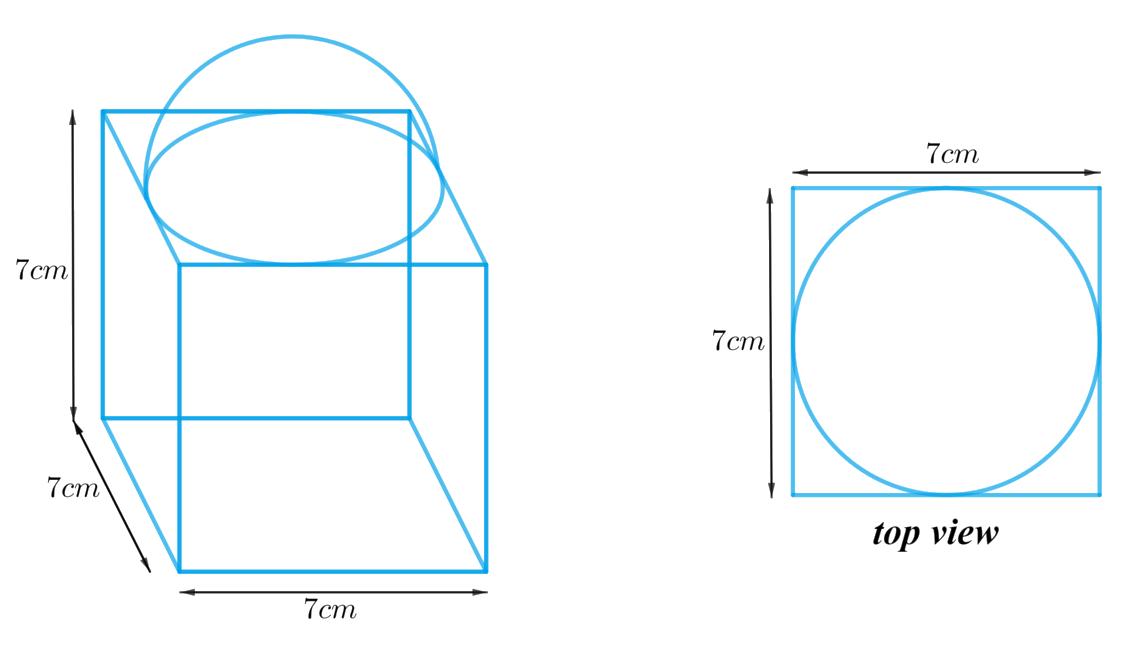From the figure its clear that the greatest diameter the hemisphere can have is equal to the cube’s edge. otherwise a part of hemisphere’s rim (circumference of its circular base) will lie outside the top part of the cube.

As it’s clear from the top view of the figure that the top part of the cube is partially covered by hemispherical part.

Total surface area of the solid $$=$$ Surface Area of the cubical part $$-$$ Area of base of hemispherical part $$+$$ CSA of the hemispherical part

We will find the total area of the solid by using formulae;
CSA of the hemisphere $$= 2\pi {r^2}$$
Area of the base of the hemisphere $$= \pi {r^2}$$
where $$r$$ is the radius of the hemisphere

Surface area of the cube  $$= 6{l^2}$$
where $$l$$ is the length of the edge of the cube.

Steps:

Length of the edge of the cube,  $$l = 7 \rm cm$$

From the figure it’s clear that the greatest diameter the hemisphere can have is equal to the cube’s edge
Diameter of the hemisphere,  $$d = l = 7 \rm cm$$
Radius of the hemisphere, \begin{align}r = \frac{d}{2} = \frac{7}{2} \rm cm\end{align}

Total surface area of the solid $$=$$ Surface area of the cubical part $$–$$ Area of the base of the
hemispherical part $$+$$ CSA of the hemispherical part

\begin{align}&= 6{l^2} - \pi {r^2} + 2\pi {r^2}\\&= 6{l^2} + \pi {r^2}\\&= \!6 \!\times \! {\left( {7\rm {cm}} \right)^2} \!+ \! \frac{{22}}{7} \times \! {\left( {\frac{7}{2} \rm {cm}} \right)^2}\\&= 6 \times 49{ \rm c{m^2}} + \frac{{22}}{7} \times \frac{{49}}{4}{ \rm c{m^2}}\\&= 294{ \rm c{m^2}} + 38.5{ \rm c{m^2}}\\&= 332.5{ \rm c{m^2}}\end{align}

## Chapter 13 Ex.13.1 Question 5

A hemispherical depression is cut out from one face of a cubical wooden block such that the diameter $$l$$ of the hemisphere is equal to the edge of the cube. Determine the surface area of the remaining solid.

### Solution

What is  known?

Diameter $$l$$ of the hemisphere is equal to the edge of the cube.

What is unknown?

The surface area of the remaining solid.

Reasoning:

We can create the figure of the solid as per given information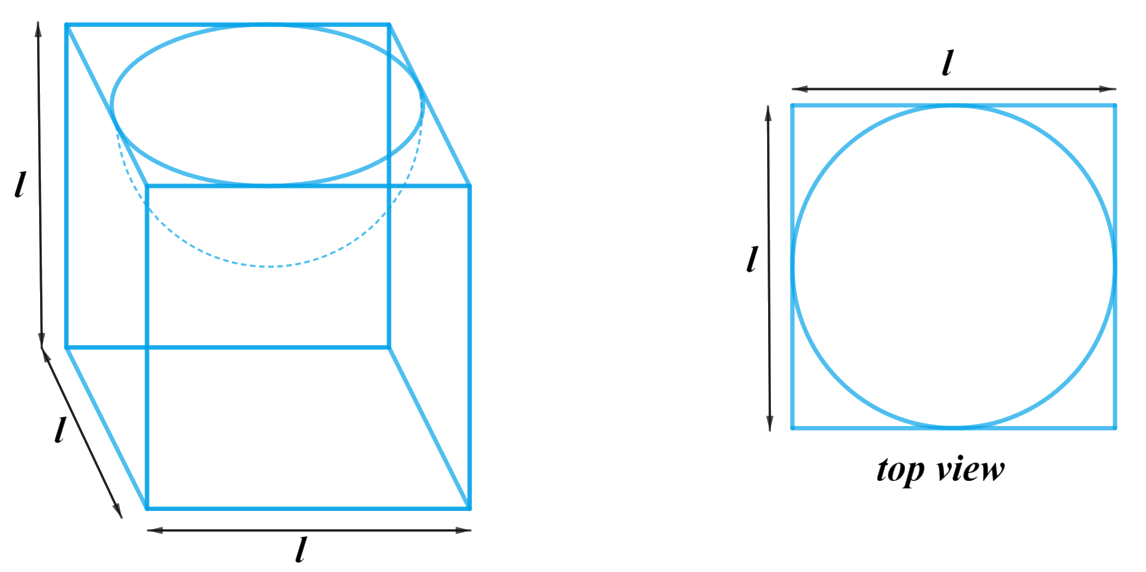From the figure it’s clear that the surface area of the remaining solid includes TSA of the cube, CSA of the hemisphere and excludes base of the hemisphere.

Surface area of the remaining solid $$=$$ TSA of the cubical part$$+$$ CSA of the hemisphericalpart $$–$$ Area of the base of the hemispherical part

We will find the remaining area of the solid by using formulae;
TSA of the cube  $$= 6{l^2}$$
where $$l$$ is the length of the edge of the cube

CSA of the hemisphere  $$= 2\pi {r^2}$$
Area of the base of the hemisphere $$= \pi {r^2}$$
where $$r$$ is the radius of the hemisphere

Steps:

Diameter of the hemisphere $$=$$ Length of the edge of the cube  $$= l$$
Radius of the hemisphere,  $$r = \frac{l}{2}$$

Surface area of the remaining solid $$=$$ TSA of the cubical part $$+$$ CSA of the hemisphericalpart $$–$$ Area of the base of the hemispherical part

\begin{align}&= 6{l^2} + 2\pi {r^2} - \pi {r^2}\\&= 6{l^2} + \pi {r^2}\\&= 6{l^2} + \pi {\left( {\frac{l}{2}} \right)^2}\\&= 6{l^2} + \frac{{\pi {l^2}}}{4}\\&= \frac{1}{4}{l^2}\left( {\pi + 24} \right)\end{align}

## Chapter 13 Ex.13.1 Question 6

A medicine capsule is in the shape of cylinder with two hemispheres stuck to each of its ends (see Fig. 13.10). The length of the entire capsule is $$14 \,\rm{mm}$$ and the diameter of the capsule is $$5 \,\rm{mm}$$. Find its surface area.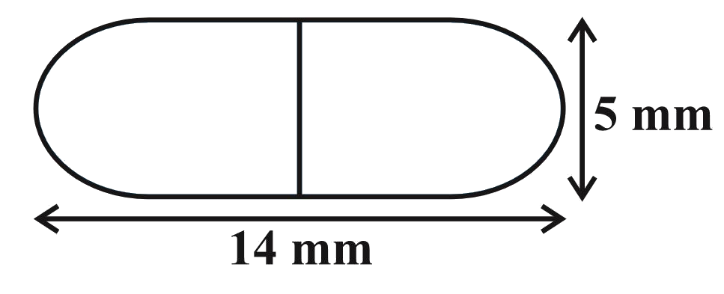### Solution

What is known?

A medicine capsule is in the shape of a cylinder with two hemispheres stuck to its ends. The length of the entire capsule $$=14 \,\rm{mm}$$, diameter of the capsule $$=5 \,\rm{mm}$$.

What is the unknown?

The surface area of the capsule.

Reasoning:

Since the capsule is in shape of a cylinder with $$2$$ hemispheres stuck to its ends,

Diameter of the capsule $$=$$ diameter of its cylindrical part $$=$$ diameter of its hemispherical part.

From the figure, it’s clear that the capsule has the curved surface of two hemispheres and the curved surface of a cylinder.

Surface area of the capsule $$= 2 \times$$ CSA of hemispherical part $$+$$ CSA of cylindrical part

We will find the surface area of the capsule by using formulae;
CSA of the hemisphere  $$= 2\pi {r^2}$$
where $$r$$ is the radius of the hemisphere
CSA of the cylinder $$= 2\pi rh$$
where $$r$$ and $$h$$ are radius and height of the cylinder respectively.

Length of the cylindrical part $$=$$ Length of the capsule $$- 2 \times$$ radius of the hemispherical part

Steps:

Diameter of the capsule,  $$d = 5 \rm mm$$
Radius of the hemisphere, \begin{align}r = \frac{d}{2} = \frac{5}{2} \rm mm\end{align}
Radius of the cylinder,  \begin{align}r = \frac{5}{2} \rm mm\end{align}
Length of the cylinder = Length of the capsule$$- 2 \times$$ radius of the hemisphere

$h = 14 \rm mm - 2 \times \frac{5}{2}mm = 9mm$

Surface area of the capsule$$- 2 \times$$ CSA of hemispherical part $$+$$ CSA of cylindrical part

\begin{align}& = 2 \times 2\pi {r^2} + 2\pi rh\\ &= 2\pi r\left( {2r + h} \right)\\ &= \begin{bmatrix} 2 \times \frac{{22}}{7} \times \frac{5}{2} \rm {mm} \times \\ \left( {2 \times \frac{5}{2}\rm {mm} + 9\rm {mm}} \right) \end{bmatrix} \\&= \frac{{110}}{7} \rm{mm} \times 14{mm}\\&= 220 \rm {m{m^2}}\end{align}

## Chapter 13 Ex.13.1 Question 7

A tent is in the shape of a cylinder surmounted by a conical top. If the height and diameter of the cylindrical part are $$2.1\,\rm{ m}$$ and $$4 \,\rm{m}$$ respectively, and the slant height of the top is $$2.8 \,\rm{m}$$, find the area of the canvas used for making the tent. Also, find the cost of the canvas of the tent at the rate of Rs $$500 \;\rm{per}\, \rm{m}^2$$. (Note that the base of the tent will not be covered with canvas.)

### Solution

What is  known?

The height and diameter of the cylindrical part are $$2.1 \rm m$$ and $$4 \rm m$$ respectively, and the slant height of the conical top is $$2.8 \rm m$$. The rate of the canvas of the tent is $$₹ 500$$ per $$\rm m^2$$.

What is unknown?

Area and cost of the canvas used for making the tent

Reasoning:

We can create the figure of the tent as per given information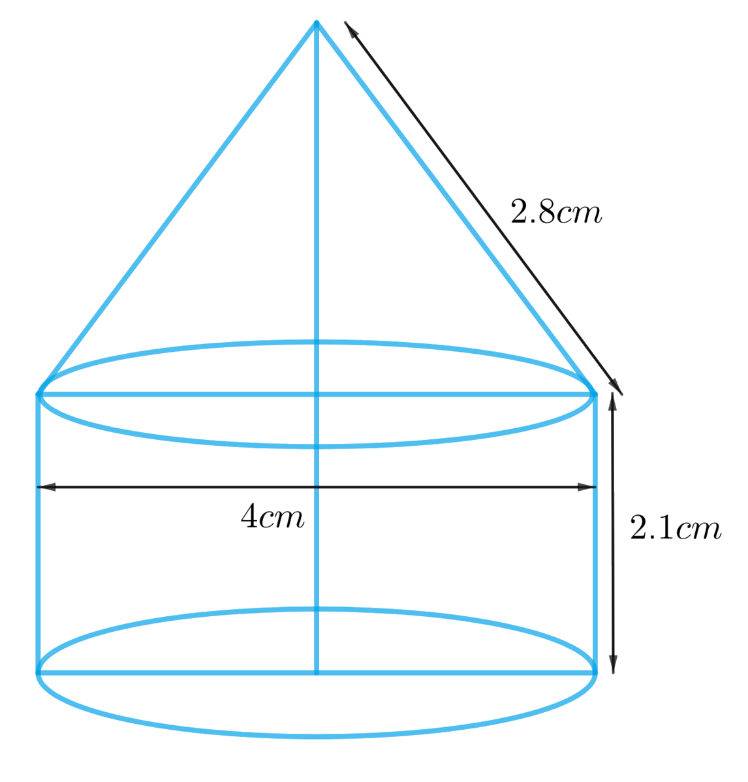From the figure it’s clear that, diameter of the cylindrical part is equal to the diameter of the conical part as the cylindrical part is surmounted by the conical part.

Since its given that the base of the tent will not be covered by the canvas then the base of the cylindrical part of the tent is not included to get the area of the canvas required.

Visually, the surface of the tent includes the curved surface of the cylindrical part and curved surface of the conical part only.
Area of the canvas used for making the tent is the surface area of the tent.

Area of the canvas used $$=$$ CSA of the cylindrical part $$+$$ CSA of the conical part

We will find the area of the canvas by using formulae;
CSA of the cone $$= \pi rl$$
where $$r$$ and $$l$$ are radius and slant height of the cone respectively.
CSA of the cylinder  $$= 2\pi rh$$
where $$r$$ and $$h$$ are radius and height of the cylinder respectively.

Cost of the canvas of the tent $$=$$ Area of the canvas $$\times$$ Rate of the canvas

Steps:

Given

Height of the cylindrical part $$h$$ $$= 2.1 \,\rm{m}$$

Diameter of the cylindrical part, $$d= 4 \,\rm{m}$$

Radius of the cylindrical, \begin{align}r = \frac{d}{2} = \frac{{4 \rm m}}{2} = 2 \rm m\end{align}

Radius of the cone,$$r = 2 \rm m$$
Slant height of the cone,  $$l = 2.8 \rm m$$

Area of the canvas used $$=$$ CSA of the cylindrical part $$+$$ CSA of the conical part

\begin{align}&= 2\pi rh + \pi rl\\&= \pi r\left( {2h + l} \right)\\&= \frac{{22}}{7}\! \times\! 2 \rm m \!\times\! ( 2 \!\times\! 2.1 \rm m \!+\! 2.8 \rm m) \\&= \frac{{44}}{7} \rm m \times 7m\\&= 44 \rm {m^2}\end{align}

Cost of the canvas of the tent $$=$$ Area of the canvas $$\times$$ Rate of the canvas

\begin{align}&= 44 \rm {m^2} \times ₹\,500/{m^2}\\&= ₹ 22000\end{align}

## Chapter 13 Ex.13.1 Question 8

From a solid cylinder whose height is $$2.4 \,\rm{cm}$$ and diameter $$1.4\,\rm{ cm}$$, a conical cavity of the same height and same diameter is hollowed out. Find the total surface area of the remaining solid to the nearest $$\rm{cm}^2$$.

### Solution

What is known?

A solid cylinder of height $$2.4 \,\rm{cm}$$ and diameter $$1.4\,\rm{ cm}$$ from which a conical cavity of the same height and same diameter is hollowed out.

What is unknown?

Total surface area of the remaining solid.

Reasoning:

We can create the figure of the solid as per given information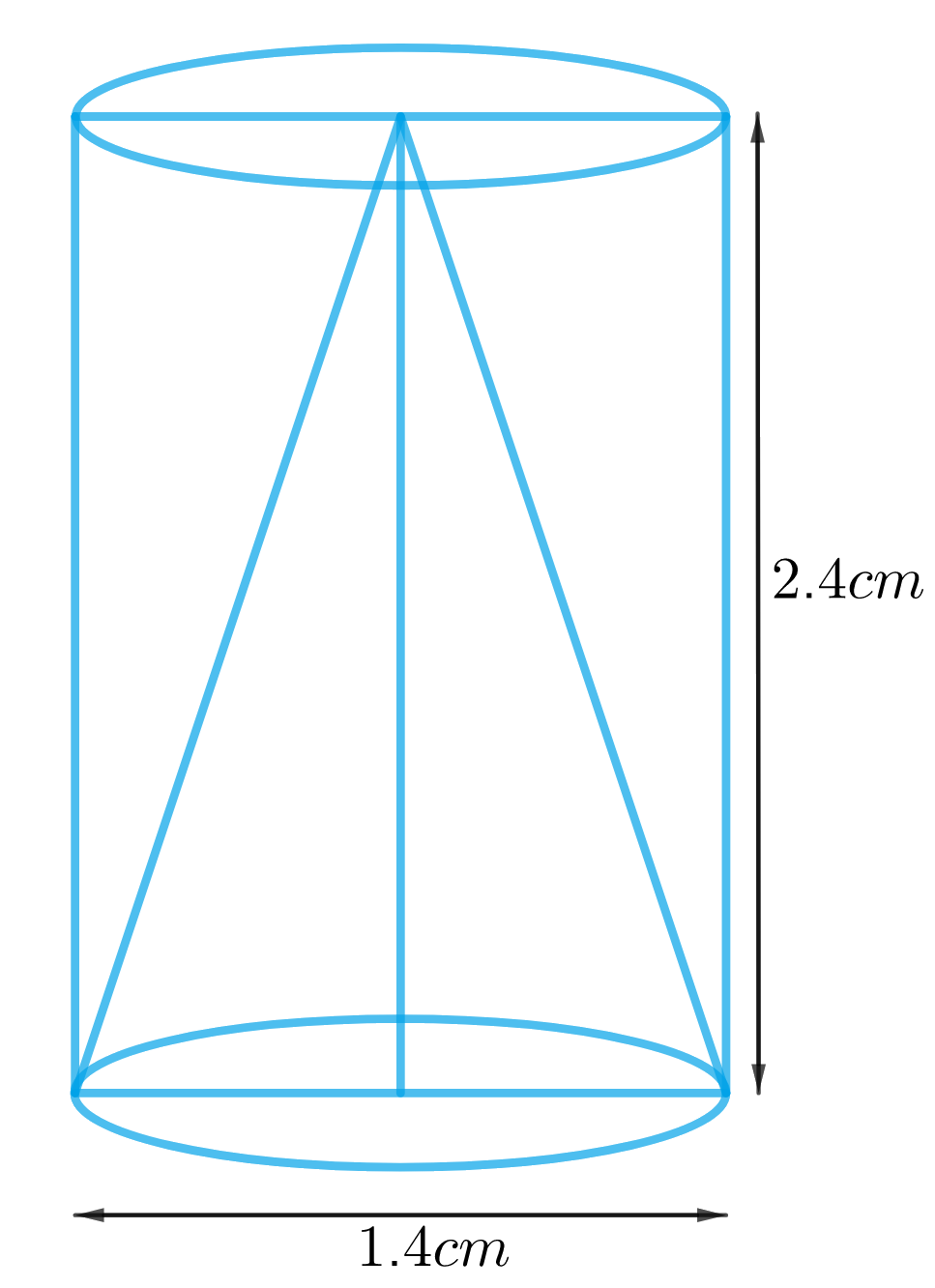Since the conical cavity of the same diameter has been hollowed out, its clear that one of the bases of the cylinder is not included in total surface area of solid.

TSA of the remaining solid $$=$$ CSA of the cylindrical part $$+$$ CSA of conical part $$+$$ Area of a cylinderical base

We will find the area of the remaining solid by using formulae;

CSA of the cylinder  $$= 2\pi rh$$
Area of the base of the cylinder  $$= \pi {r^2}$$

where $$r$$ and $$h$$ are radius and height of the cylinder respectively.
CSA of the cone $$= \pi rl$$

Slant height of the cone, $$l = \sqrt {{r^2} + {h^2}}$$
where $$r, h$$ and $$l$$ are radius, height and slant height of the cone respectively.

Steps:

Height of the cylinder $$=$$ Height of the cone $$= h = 2.4 \rm cm$$

Diameter of the cylinder $$=$$ diameter of the cone $$= d = 1.4 \rm cm$$

Radius of the cylinder $$=$$ radius of the cone \begin{align} = r = \frac{{1.4 \rm cm}}{2} = 0.7 \rm cm\end{align}

Slant height of the cone,$$l = \sqrt {{r^2} + {h^2}}$$

\begin{align}l &= \sqrt {{{\left( {0.7\rm cm} \right)}^2} + {{\left( {2.4cm} \right)}^2}} \\ &= \sqrt {0.49 \rm c{m^2} + 5.76c{m^2}} \\&= \sqrt {6.25 \rm c{m^2}} \\&= 2.5 \rm cm\end{align}

TSA of the remaining solid $$=$$ CSA of the cylindrical part $$+$$ CSA of conical part $$+$$Area of the cylindrical base

\begin{align}&= 2\pi rh + \pi rl + \pi {r^2}\\& = \pi r\left( {2h + l + r} \right)\\&= \!\!\frac{{22}}{7}\! \!\times\! 0.7 \rm cm \!\times\!\! ( 2 \!\times\! 2.4 \rm cm \!+ \! 2.5 \rm cm \!+\! 0.7cm ) \\&= 2.2 \rm cm \times 8cm\\&= 17.6 \rm c{m^2}\end{align}

Hence, the total surface area of the remaining solid to the nearest $$\rm cm^2$$ is $$18 \, \rm cm^2.$$

## Chapter 13 Ex.13.1 Question 9

A wooden article was made by scooping out a hemisphere from each end of a solid cylinder, as shown in Fig. 13.11. If the height of the cylinder is $$10 \rm{cm}$$, and its base is of radius $$3.5 \rm{cm,}$$find the total surface area of the article.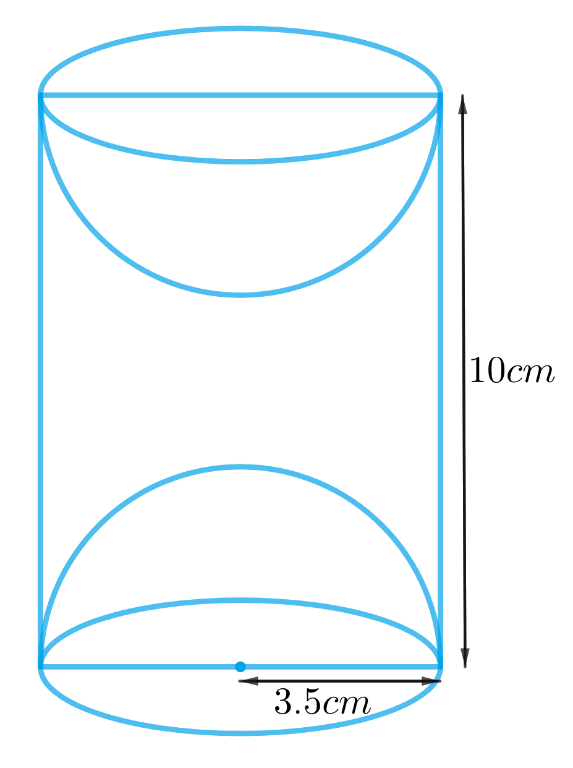### Solution

What is known?

The height of the cylinder is $$10 \rm{cm}$$, and radius of its base is $$3.5 \rm{cm}$$

What is unknown?

Total surface area of the article.

Reasoning:

From the figure it’s clear that radius of the hemispheres scooped out is same as the radius of base of the cylinder since both the hemispheres are of equal radius.
So, total surface area of the article only includes the CSA of both the hemispheres and the cylinder.

TSA of the article $$= 2 \times$$ CSA of the hemispherical part $$+$$ CSA of the cylindrical part

We will find the TSA of the article by using formulae;

CSA of the hemisphere  $$= 2\pi {r^2}$$

where r is the radius of the hemisphere

CSA of the cylinder  $$= 2\pi rh$$

where $$r$$ and $$h$$ are radius and height of the cylinder respectively.

Steps:

Height of the cylinder  $$= h = 10 \rm cm$$

Radius of the cylinder $$=$$ radius of the hemisphere $$= r = 3.5 \rm cm$$

TSA of the article $$=$$ $$2 \times$$  CSA of the hemispherical part $$+$$ CSA of the cylindrical part

\begin{align}&= 2 \times 2\pi {r^2} + 2\pi rh\\&= 2\pi r\left( {2r + h} \right)\\&= 2\! \times \!\!\frac{{22}}{7}\!\! \times \!3.5 \rm cm \!\times\! ( 2 \!\times\! 3.5 \rm cm\!+ \!10 \rm cm )\\&= 22 \rm cm \!\times \!17 \rm cm\\&= 374 \rm c{m^2}\end{align}

Download SOLVED Practice Questions of NCERT Solutions For Class 10 Maths Chapter 13 Exercise 13.1 for FREE
Ncert Class 10 Exercise 13.1
Ncert Solutions For Class 10 Maths Chapter 13 Exercise 13.1

Related Sections
Related Sections
Instant doubt clearing with Cuemath Advanced Math Program